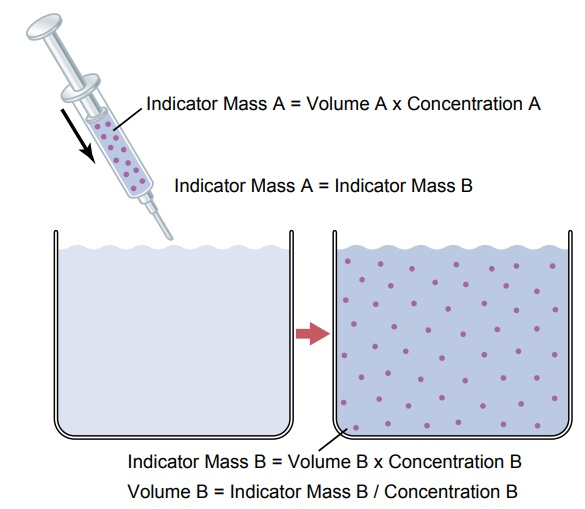Home | | Medical Physiology | Measurement of Fluid Volumes in the Different Body Fluid Compartments-The Indicator-Dilution Principle

# Measurement of Fluid Volumes in the Different Body Fluid Compartments-The Indicator-Dilution PrincipleThe volume of a fluid compartment in the body can be measured by placing an indicator substance in the compartment, allowing it to disperse evenly through-out the compartment’s fluid, and then analyzing the extent to which the substance becomes diluted.

Measurement of Fluid Volumes in the Different Body Fluid Compartments—The Indicator-Dilution Principle

The volume of a fluid compartment in the body can be measured by placing an indicator substance in the compartment, allowing it to disperse evenly through-out the compartment’s fluid, and then analyzing the extent to which the substance becomes diluted. Figure 25–4 shows this “indicator-dilution” method of meas-uring the volume of a fluid compartment, which is based on the principle of conservation of mass. This means that the total mass of a substance after disper-sion in the fluid compartment will be the same as the total mass injected into the compartment.In the example shown in Figure 25–4, a small amount of dye or other substance contained in the syringe is injected into a chamber, and the substance is allowed to disperse throughout the chamber until it becomes mixed in equal concentrations in all areas.

Then a sample of fluid containing the dispersed sub-stance is removed and the concentration is analyzed chemically, photoelectrically, or by other means. If none of the substance leaks out of the compartment, the total mass of substance in the compartment (Volume B x Concentration B) will equal the total mass of the substance injected (Volume A x Con-centration A). By simple rearrangement of the equation, one can calculate the unknown volume of chamber B asVolume B= [Volume A x Concentration A]/ Concentration B

Note that all one needs to know for this calculation is (1) the total amount of substance injected into the chamber (the numerator of the equation) and (2) the concentration of the fluid in the chamber after the substance has been dispersed (the denominator). For example, if 1 milliliter of a solution containing 10 mg/ml of dye is dispersed into chamber B and the final concentration in the chamber is 0.01 mil-ligram for each milliliter of fluid, the unknown volume of the chamber can be calculated as follows:This method can be used to measure the volume of virtually any compartment in the body as long as (1) the indicator disperses evenly throughout the com-partment, (2) the indicator disperses only in the com-partment that is being measured, and (3) the indicator is not metabolized or excreted. Several substances can be used to measure the volume of each of the differ-ent body fluids.

Study Material, Lecturing Notes, Assignment, Reference, Wiki description explanation, brief detail

Related Topics